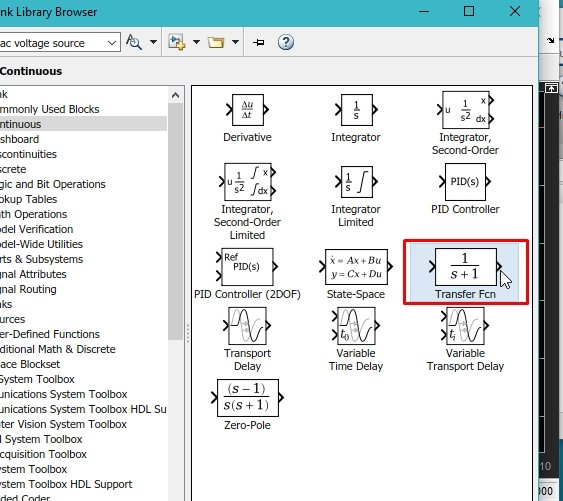# How to use Lags and delay in simulink : tutorial 9

In this tutorial, I will explain you the working of lags and delays in Simulink. Lags and delays seem to be a similar term but in fact they are not. In this tutorial we will discuss that particular difference between lags and delays. In simulink there are multiple blocks that can do the delay operation with a little distinction in their functionality. We will also discuss that distinction in this tutorial along with the working and implementation of each block. I will provide you with a comprehensive example regarding the working of delays (two types from the simulink library browser) i.e. Delay block and Transport delay block also I will explain the working of lags in the output or response of a system and their difference.

### How to use Lags and delay in simulink

Figure 1: Library browser

Figure 2: Sources blocks

Figure 3: Step

Figure 5: Sinks

Figure 6: Step time

Figure 7: Diagram

Figure 8: Running

Figure 9: Step input

Figure 10: Delay block

Figure 11: Input ports

Figure 12: Diagram

Figure 13: Delayed output

Figure 14: Changed delay

Figure 15: Continuous block

Figure 16: Transport delay

Figure 17: Delay time

Figure 18: Number of ports

Figure 19: Diagram

Figure 20: Delayed outputs

• The red wave in the above graph is the input of the step block, the yellow is the output of the delay block and the blue is the output of the transport delay block. The difference in the outputs of the delayed blocks is visible from the graphs shown in the figure above. The small slope of in the transport delay blocks’ output is also referred as a lag which occurs in all the physical circuits of real time. Lets’ discuss this lag operation further more using another different block. From the continuous section of the library browser select the transfer function block as shown in the figure below,Figure 21: Transfer function block

Figure 22: Diagram

Figure 23: Legends

Figure 24: Output

• The lagged output is the red one, and is same as the input but it is showing the real time effect of the normal step input function.